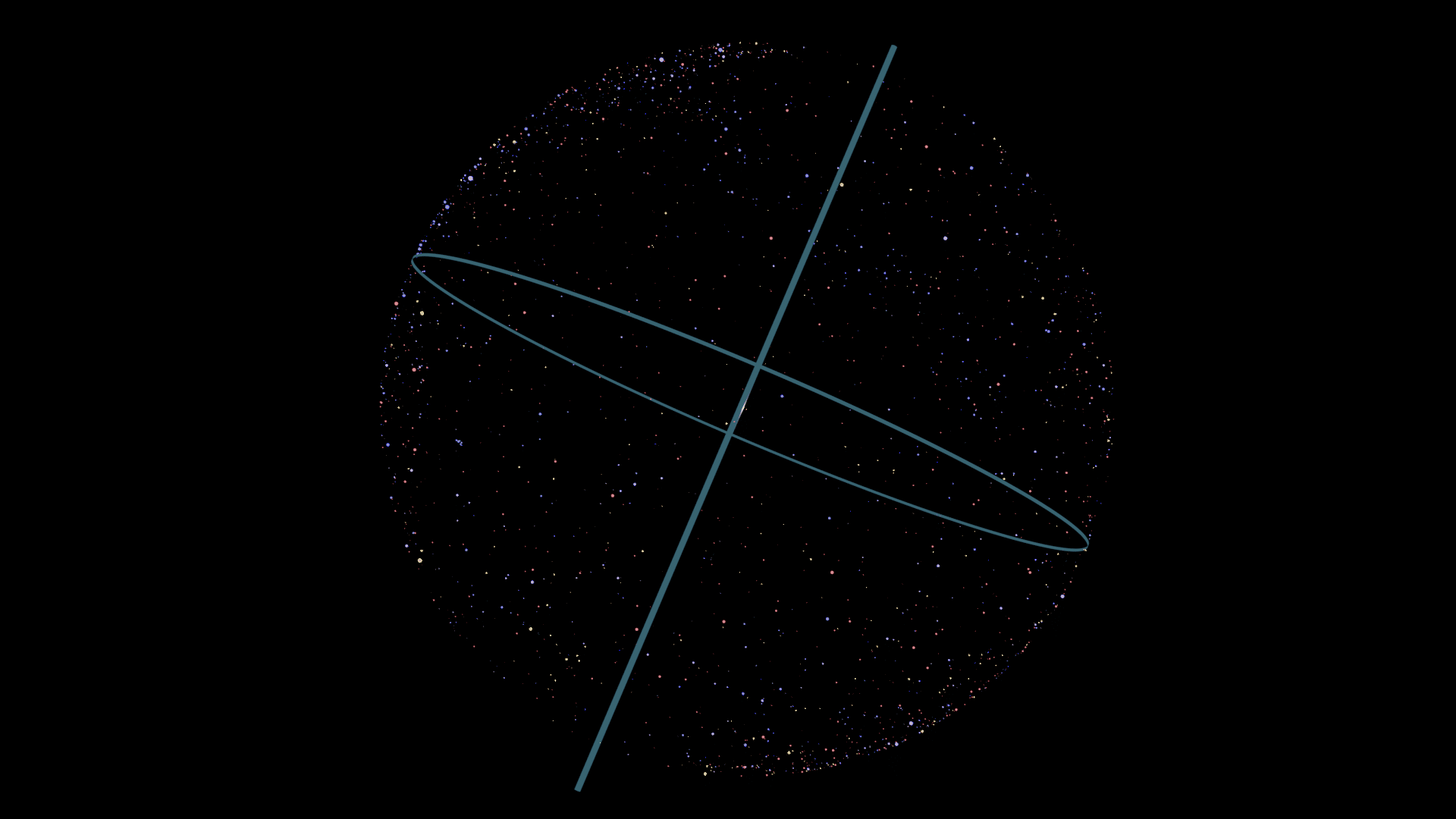# Coordinates in The Night Sky

The position of stars can be described by coordinates that resemble that way we describe positions on the Earth’s surface. The concept is that we imagine that the stars are all on some huge sphere that the Solar System is well inside, with the centre being the Earth. This massive sphere is called the Celestial Sphere. An important thing to remember is that the sphere is not real it is just a way for us humans to conceptualise the night sky so that we can describe the positions of objects, mainly so other people can find them. For most of human history we have had no idea just how far away the stars are – even the distance to our own star, the Sun, was not well known until the late 1700s. Despite this a common way of describing where stars could be found was developed, the distances would come later.If all of the visible stars were placed on a sphere surrounding the Solar System, this is what it might look like from the outside.

### Celestial Poles

An observer not exactly on the equator they will observe the stars in the night sky circling around one point. If they are on the equator they would see the stars circling two points on opposite horizons. The point that the stars are circling is an extension of the Earth’s axis on the Celestial Sphere. In the Southern Hemisphere this is called the South Celestial Pole, or SCP for short.

### Declination

This point is called the North Celestial Pole in the Northern Hemisphere. These points are given a position on the Celestial Sphere which is based on the angle from the Equator to the axis, 90 degrees. So in the North this is +90 degrees and in the South this is -90 degrees. This coordinate is called declination and it’s a bit like latitude. So that’s the up and down coordinate, what about the left and right.

### Celestial Equator

The coordinate that is similar to longitude for identifying positions on the Celestial Sphere is a bit trickier to figure out. Imagine the equator of the Earth extended all the way to the Celestial Sphere, this is the Celestial Equator.The Earth’s axes and equator extended out to the Celestial Sphere marking the Celestial Poles and the Celestial Equator.

### Vernal Equinox

There is an angle between the Earth’s axis and the plane of rotation around the Sun of 23.5 degrees. The Sun appears to take a path in the sky that crosses that Celestial Equator twice per year. This crossing point is exactly at the equinoxes and the point, as viewed from the Northern Hemisphere, where the Sun appears to cross the Celestial Equator from the South to the North is called the Vernal Equinox, the Spring Equinox.

### Right Ascension

That point on the Celestial Equator is important because that is where we measure the left and right coordinate. That point is the zero point for the scale and it works like longitude but it’s in hours. Each hour is the equivalent of 15 degrees. This set up means that you can always find an object because it doesn’t matter what the Earth is doing. As long as you can find the reference point to start measuring the hour from you’ll be able to find anything.

This measure is called Right Ascension and is used with declination to describe any point on the Celestial Sphere.

Having this doesn’t make it easy to use. The earth is rotating so the right ascension is going to constantly change on the surface of the Earth. The Prime Meridian is the other key point in the night sky. The Prime Meridian is a great circle passing through North and the Zenith. The difference between the zero angle point on the Celestial Equator and the Prime Meridian is called the hour angle . This will help you find the object relative to the position of the prime meridian.

Scroll to Top